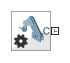# Mechanism Configuration

Mechanism-wide simulation and mechanical parameters

## Library

Utilities

•## Description

This block provides mechanical and simulation parameters to a mechanism, i.e., a self-contained group of interconnected Simscape™ Multibody™ blocks. Parameters include gravity and a linearization delta for computing numerical partial derivatives during linearization. These parameters apply only to the target mechanism, i.e., the mechanism that the block connects to.

The Mechanism Configuration block is optional. If you omit it, the gravitational acceleration vector is set to zero. Use only one instance of this block per mechanism, setting uniform gravity to `None` if that mechanism contains one or more Gravitational Field blocks.

## Parameters

Uniform Gravity

Type of gravitational acceleration vector in effect at the target mechanism. Options include:

• `None` — Specify zero gravity. The block automatically applies the gravitational acceleration vector [0 0 0] to the target mechanism. If the mechanism contains one or more Gravitational Field blocks, you must select this option.

• `Constant` — Specify a gravitational acceleration vector that remains constant in space and in time. Selecting this option exposes an additional parameter, Gravity. If the target mechanism contains one or more Gravitational Field blocks, you must select `None` instead.

• `Time-Varying` — Specify a gravitational acceleration vector that remains constant in space but varies in time. Selecting this option exposes a physical signal port. Use that port to specify the time-varying gravitational acceleration vector. If the target mechanism contains one or more Gravitational Field blocks, you must select `None` instead.

Gravity

Nominal acceleration vector due to gravity. The block resolves this vector in the mechanism World frame. The default vector is ```[0 0 –9.80665]``` m/s^2.

Linearization Delta

Perturbation value for computing numerical partial derivatives during linearization. The default value is `0.001`.

## Ports

PortDescription
CFrame port that identifies the target mechanism to which the block parameters apply.
gPhysical signal port through which you specify a time-varying gravity vector.

## Version History

Introduced in R2012a